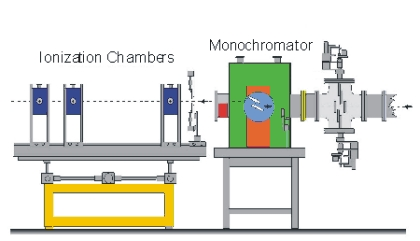# Interdisciplinary Nanoscience Center# X-ray absorption spectroscopy

## X-ray absorption spectroscopy.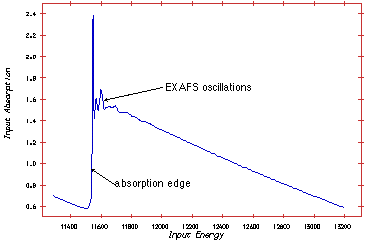Extended X-ray Absorption Fine Structures (EXAFS). The technique exploits the fact that X-ray absorption is unique for each atom in its chemical surroundings. The absorption is measured as a function of wavelength (l) or energy (E) of the X-ray radiation.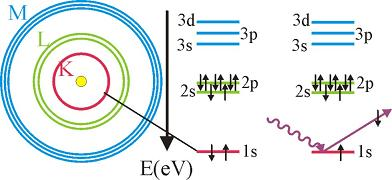The excited atom can relax through several mechanisms, giving rise to fluorescence, Auger electrons or secondary electrons.

Thus, the absorption spectra as a function of energy photon energy has spikes each time the photon energy reaches the binding energy of a given shell.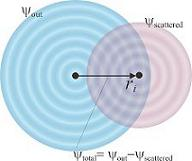The cross-section for absorption can be expressed using Fermi's golden rule:

W ~ < phi final | H' | phi initial >

H' is the perturbation introduced by the electro-magnetic field of the photon. Phi initial is the initial state, where the electron is bound in the atomic potential. Phi final is the final state of the electron. If the atom has no neighbours it is considered to be a spherical wave. A neighbouring atom will cause scattering and, thereby, modify the final state of the electron. The result of differing final states is the EXAFS spectrum.

Taking various circumstances into consideration the a master formula can be written: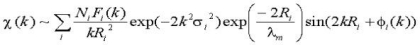This describes the absorption as a function of the length of the wavevector |k| = 2p/l. The sum considers the number of atoms Ni with a distance Ri to the centre atom. Fi(k) is the scattering amplitude. Si is the Debye-Waller factor describing the motion of neighbouring atoms. lm is the mean path of the electron, the length the electron travels before it is scattered. The sinus term gives the phase difference, the first term is the phase from the propagating wave, whereas the second term is a phase function related to the changing potential. From the expression it is possible to derive atomic distances and the co-ordination numbers.

## Measurements

The measuring principle is simple. The energy (wavelength) is scanned in a range just below the absorption edge to around 1000 eV above the edge. Two ionisation chambers are needed; one placed in front of the sample and one behind. Often three chambers is used. The gas in the ionisation chamber is ionised by a fraction of the X-ray. The ionised gas atoms produce a current, which is measured. To obtain the EXAFS spectre the signal from chamber two is normalised to the signal from chamber one.